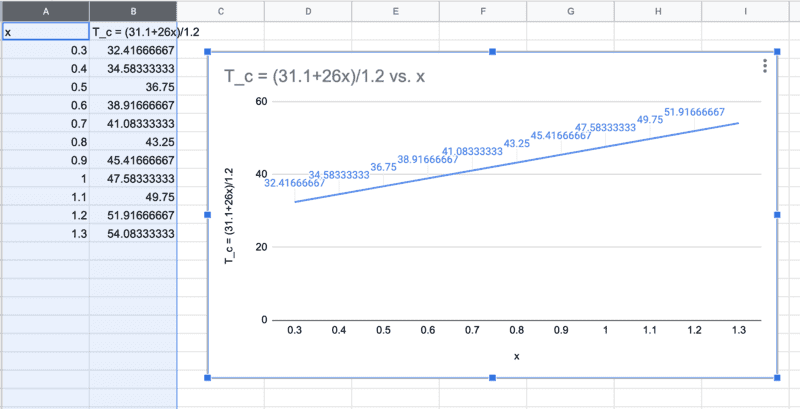# Torque problem - Hanging sign

paulimerci
Yes. Find an expression for ##R_y## containing ##x##. Then substitute ##x=1.00## m and see if you get the answer that you already got in part (b).

Correction to your work: The right chain should be ##x+0.3~##m.
Okay, if there are changes in distances does the values of ##T_c##, ##T_L##, and ##T_R## remains the same? If there is no change, then in the torque equation can I substitute for the values i calculated earlier for ##T_c##, ##T_L##, ##T_R##?

paulimerci
Okay, if there are changes in distances does the values of ##T_c##, ##T_L##, and ##T_R## remains the same? If there is no change, then in the torque equation can I substitute for the values i calculated earlier for ##T_c##, ##T_L##, ##T_R##?
$$\sum\tau= T_c \cdot d_c-Mg \cdot d_B-T_L \cdot x-T_R \cdot (x+0.30)$$
$$1.2 \cdot T_c = 27.2+ 13 \cdot x + 13 \cdot(x+0.3)$$
$$T_c = \frac {31.1 +26 \cdot x} {1.2}$$
Substitute ##T_c## in the equation of ##R_y## we get,
$$R_y = Mg +T_L+T_R-T_c$$
$$R_y = 34+13+13- \frac{31.1+26\cdot x} {1.2}$$
$$R_y = 60 - \frac{31.1+26\cdot x} {1.2}$$
have I done it right?

•kuruman
Homework Helper
Gold Member
Very good. Now you can answer parts (c) and (d) based on the equation you derived for ##R_y.##

paulimerci
Very good. Now you can answer parts (c) and (d) based on the equation you derived for ##R_y.##
Part C,
Therefore, from the equation of ##R_y## we can justify that as the distance of the sign is moved farther away from the wall, the magnitude of the force on the hinge decreases.

•Lnewqban
Homework Helper
Gold Member
Part C,
Therefore, from the equation of ##R_y## we can justify that as the distance of the sign is moved farther away from the wall, the magnitude of the force on the hinge decreases.
Correct. Now for the plot. Use a spreadsheet if you know how.

paulimerci
Correct. Now for the plot. Use a spreadsheet if you know how.••